# Euclidean Geometry

Euclidean Geometry

In this mini-lesson, we will explore the world of Euclidean geometry by understanding euclidean and non-euclidean geometry, the postulates and axioms, and how to apply them while solving problems. We will also discover interesting facts around them.

The Alexandrian Greek mathematician Euclid has contributed the whole of his life towards new inventions and discoveries in mathematics.

The mathematics community is so thankful for his work on geometry.

The "Elements" is the textbook he wrote on geometry which contains his postulates and axioms on basic geometry.

The Elements series consists of 13 books, the books starting from 1th to 4th and 6th discuss only plane geometry.

The books 5th and 7th to 10th deal with the number theory which introduced prime numbers, rational numbers, and irrational numbers.

Books 11th to 13th explains solid geometry in which he explained that the volume of the cone is one-third of the volume of the cylinder with the same height and base and he also explained how the platonic solids are constructed.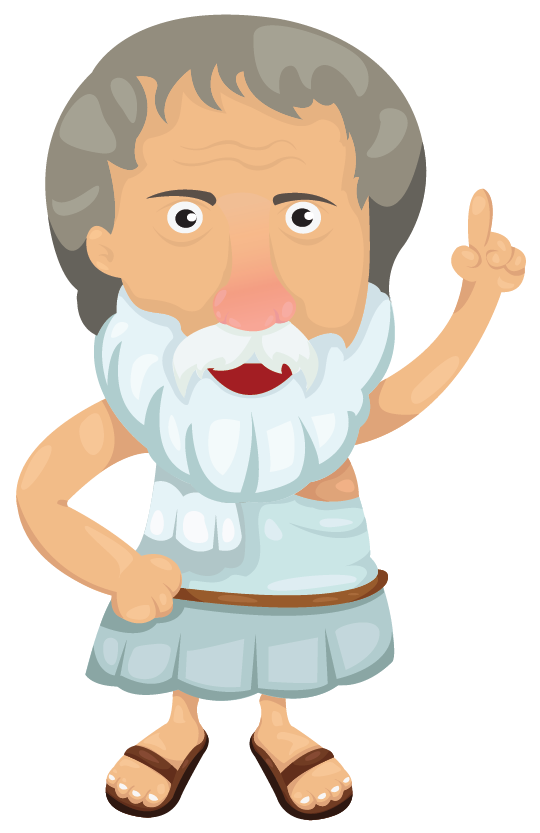Do you know that the work of Euclid is known for 2000 years and still many of us use his work?

Let's explore Euclidean geometry in more detail.

## Lesson Plan

 1 What Is Euclidean Geometry? 2 Important Notes on Euclidean Geometry 3 Thinking Out of the Box! 4 Solved Examples on Euclidean Geometry 5 Interactive Questions on Euclidean Geometry

## What Is Euclidean Geometry?

In Euclidean geometry, we study plane and solid figures based on postulates and axioms defined by Euclid. Euclid is known as the father of geometry because of the foundation laid by him. Euclid defined a basic set of rules and theorems for a proper study of geometry.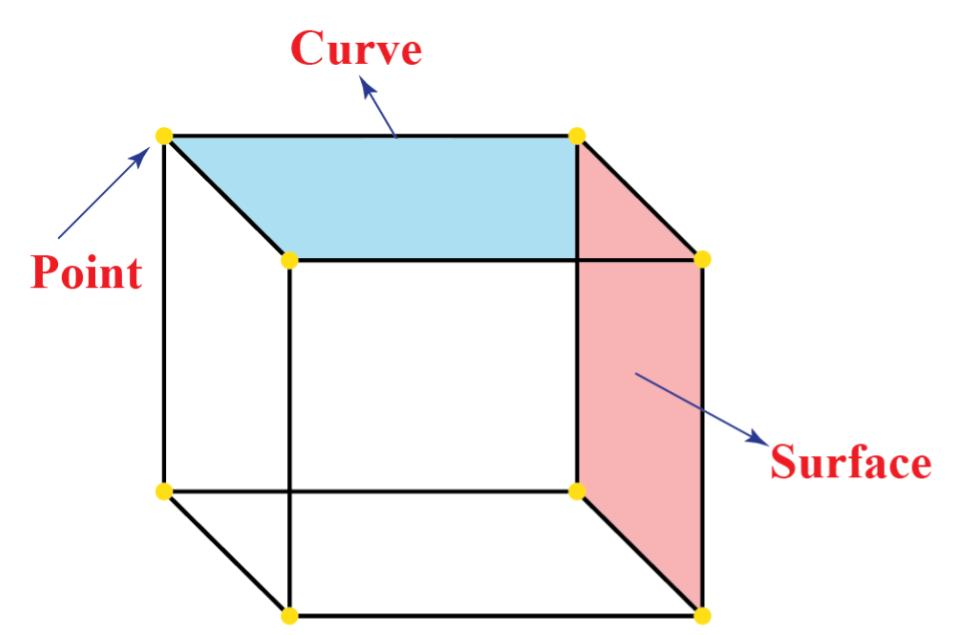The Greek mathematician Euclid provided the definitions of point, line, and plane (surface). According to Euclid, a solid shape has a shape, size, and position and it can be moved from one position to another.

The boundaries of these solids are called surfaces, these surfaces separate one part of the space from another and have no thickness.

The boundaries of these surfaces are called curves or straight lines and these straight lines end in a point. There exists no straight line. Each line that appears straight is a part of a very big circle.

When we moved down the hierarchy of the parts of solids, the dimensions of each part are also reducing by one.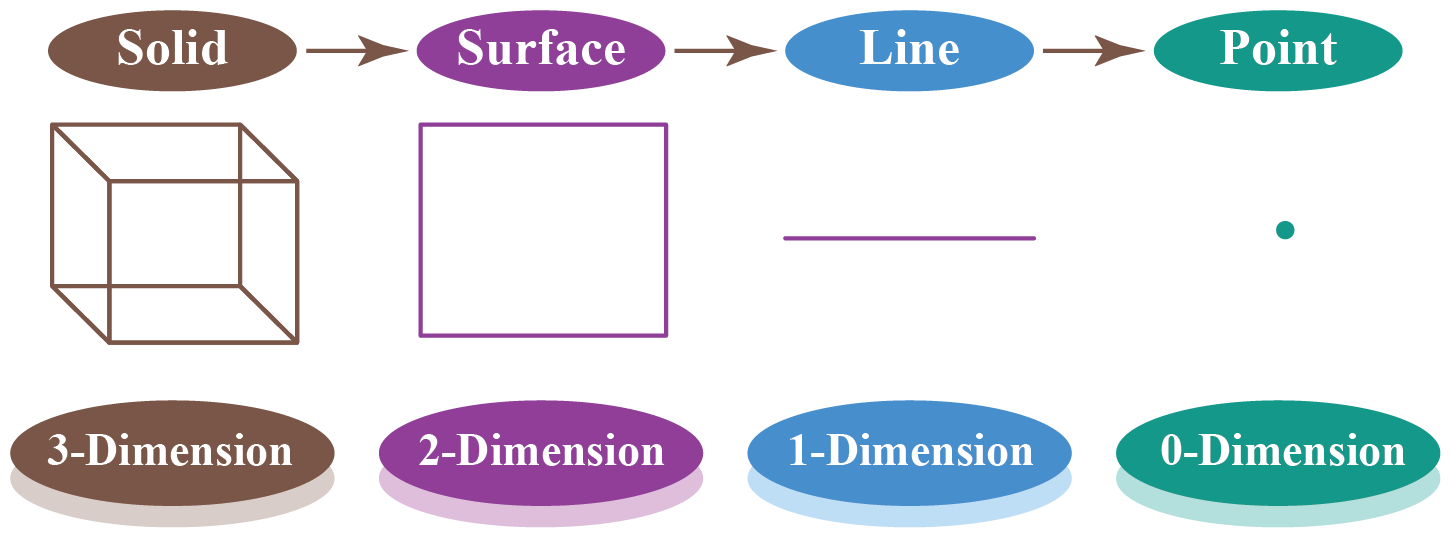In each of the three steps from solid to point, we are losing one dimension.

A solid has three dimensions, a surface has two dimensions, a line has one dimension and a point has zero dimension.

There is a branch of geometry known as Non-Euclidean geometry. Basically, it is everything that does not fall under Euclidean geometry. However, it is commonly used to describe spherical geometry and hyperbolic geometry.

### Spherical Geometry

The Greek astronomer Ptolemy wrote in his book Geography that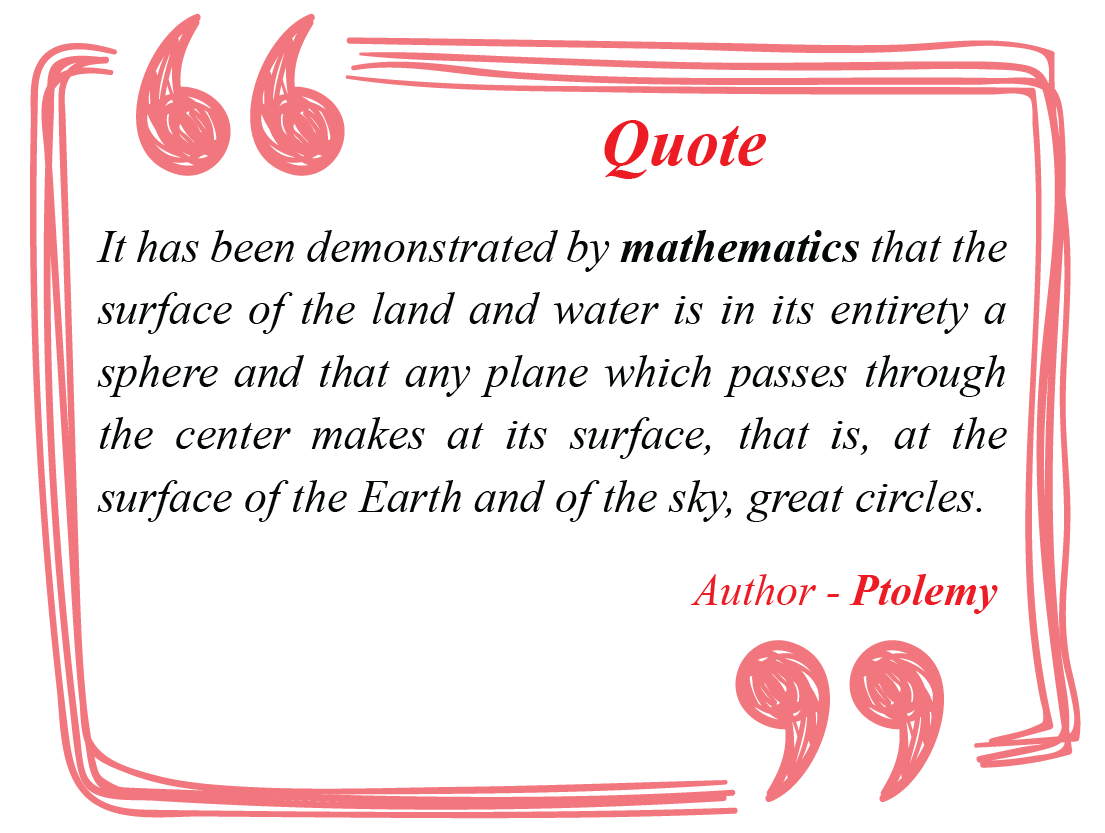We all know that the earth is spherical, but how can we project the whole of the earth on a plane surface with the help of maps?While converting the actual distance or points from spherical geometry to Euclid's geometry (Basic Geometry) we have to change actual distances, location of points, area of the regions, and actual angles.

### Hyperbolic Geometry

Euclid's postulates explain hyperbolic geometry.

The studies conducted in mid 19 century on hyperbolic geometry has proved that hyperbolic surface must have constant negative curvature, but the question of "whether any surface with hyperbolic geometry actually exists?" still arise before every researcher.

In 1868, Italian mathematician Eugenio Beltrami described a pseudosphere, that has constant negative curvature, but the pseudosphere is not a complete demonstration of hyperbolic geometry.

The below image shows a hyperbolic surface.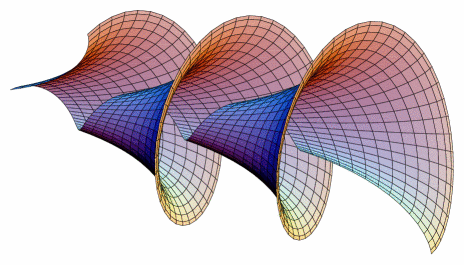## Euclid’s Postulates

The most famous Greek mathematician has proposed five postulates on geometry, these postulates are widely accepted by the mathematics community.

Let's see these postulates.

### Euclid's Postulate 1

“A straight line segment can be drawn for any two given points.”

For any two points, a line can be drawn such that it passes through both of them.

### Euclid's Postulate 2

“To produce a finite line segment continuously in a straight line.”

A line segment can be extended in any direction to form a line.

### Euclid's Postulate 3

“To describe a circle with any center and radius.”

A circle can be defined by taking any point as the center and having a specified radius.

### Euclid's Postulate 4

“That all right angles are equal.”

Any angle that measures 90° is equal to another 90° angle, irrespective of the length of the lines forming the angles.

### Euclid's Postulate 5

“If two lines are intersected by a third in such a way that the sum of the inner angles on one side is less than two right angles, then the two lines will intersect each other on that side if produced indefinitely.”

When there are two lines cut by a third line, if the sum of the interior angles is less than 180°, then the two lines will meet when extended on that side.

In the image given below, $$\angle 1 + \angle 2 < 180^{\circ}$$.

∴ Line $$m$$ and $$n$$ will meet when extended on the side of 1 and 2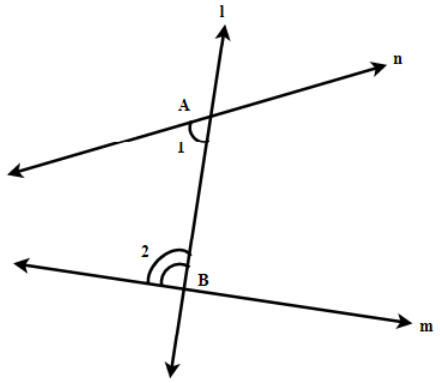Think Tank
• Does a 4th dimension exist? If yes then what do the solids of the 4th dimensions look like?

## Euclid’s Axioms

Axioms or postulates are the assumptions of the obvious universal truths. They are not proved.

Listed below are Euclid’s Axioms:

1. Things that are equal to the same thing are equal to one another.

2. If equals are added to equals, the wholes are equal.

3. If equals are subtracted from equals, the remainders are equal.

4. Things that coincide with one another are equal to one another.

5. The whole is greater than the part.

6. Things that are double of the same things are equal to one another.

7. Things that are halves of the same things are equal to one another.

## Solved Examples on Euclidean Geometry

Geometry
grade 9 | Questions Set 1
Geometry
Geometry
grade 9 | Questions Set 2
Geometry
More Important Topics
Numbers
Algebra
Geometry
Measurement
Money
Data
Trigonometry
Calculus
More Important Topics
Numbers
Algebra
Geometry
Measurement
Money
Data
Trigonometry
Calculus